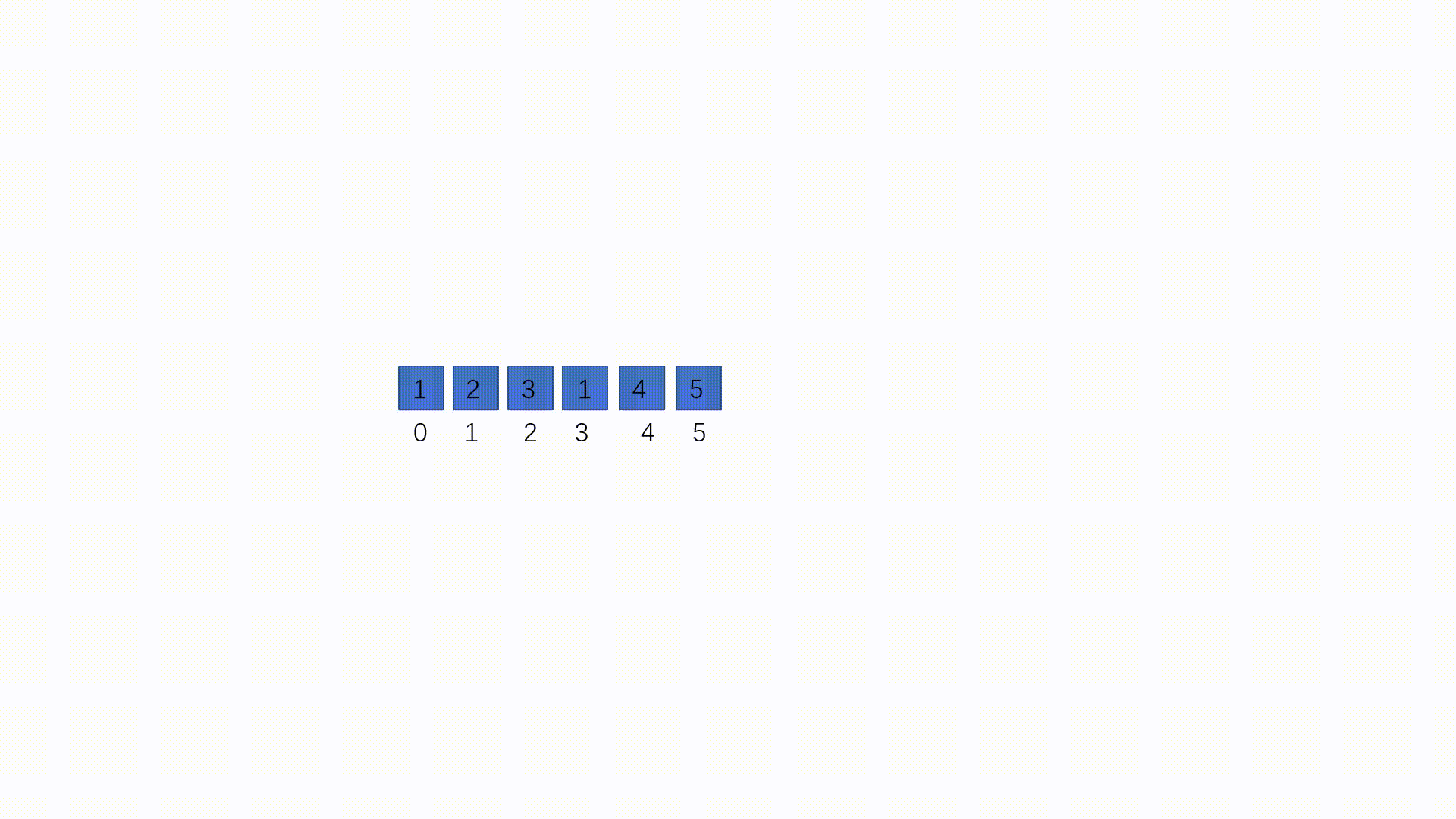# LeetCode219. 存在重复元素 II

LeetCode219. 存在重复元素 II

输入: nums = [1,2,3,1], k = 3



输入: nums = [1,0,1,1], k = 1



输入: nums = [1,2,3,1,2,3], k = 2代码实现：

//存在重复元素 II
class Leetcode219{
public static void main(String[] args){
int[] arr={1,2,3,1,2,3};
int k=2;
System.out.println(containsNearbyDuplicate(arr, k));
}
public static boolean containsNearbyDuplicate(int[] nums, int k) {
for(int i=0;i<nums.length;i++){     //遍历数组
for(int j=i+1;j<nums.length;j++){   //从第i+1个元素遍历数组
if(nums[i]==nums[j]){         //如果存在相等的元素
if(Math.abs(j-i)<=k){       //求两索引的差的绝对值是否小于等于k
return true;        //满足则返回true
}
}
}
}
return false;
}
}


©️2019 CSDN 皮肤主题: 大白 设计师: CSDN官方博客